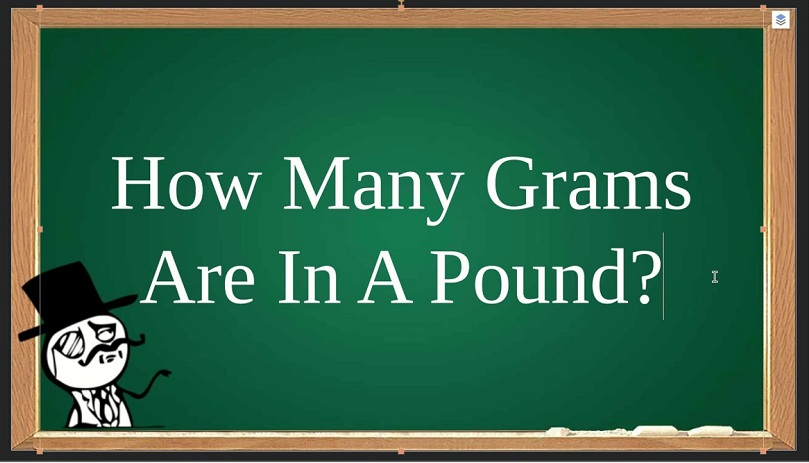Home Education How Many Grams Are In 1 Pound?

# How Many Grams Are In 1 Pound?

52There are 453.59237 grams in a pound (lb).

The gram is a metric system unit of mass. Originally defined as “the absolute weight of a volume of pure water equal to the cube of the hundredth part of a metre [1 cm³], and at the temperature of melting ice”.

A gram is a unit of weight equal to 1/1000th of a kilogram.

A pound is defined as exactly 0.45359237 kilograms.